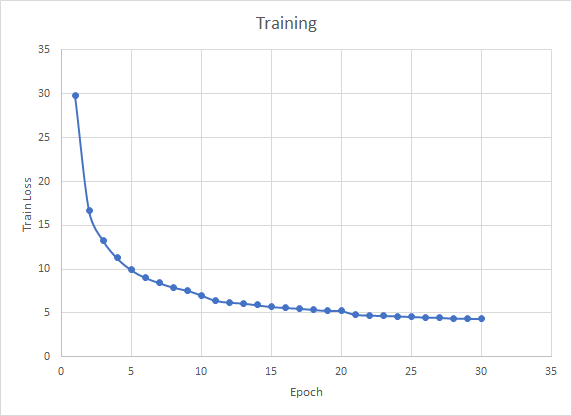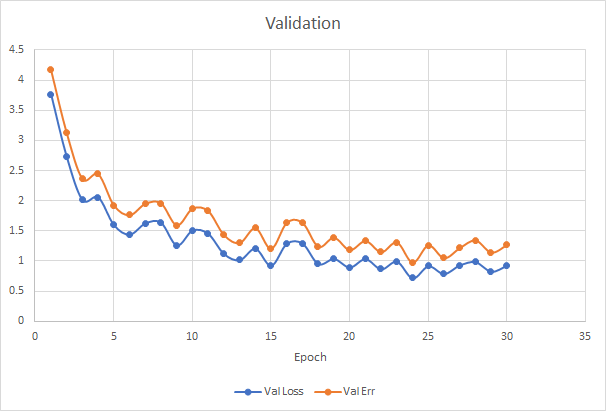# Validation loss/error curve isn't as smooth as training loss curve

Hi all,

I am training a network that perform depth estimation. My training loss/epoch curve is decreasing smoothly but my validation loss and error / epoch curves although are decreasing but is like a roller coaster ride. I am just wondering if this phenomenon normal? I can’t really seem to find a good answer elsewhere to decided to ask here.

Thank you.

Learning rate started with 0.001 and is decayed by 0.0003 after every 10 epochs.
Total epochs: 30
Batch Size: 16How large is your validation set?
If you are calculating the validation loss on fewer samples, it might explain the noise in the loss curve.

My validation set has a size of 1460 and my training set is 35454. So my validation set is about 4% of my training set. Is this size too small?

But intuitively validation is just to check if our model is converging right, so I can ignore the noise as long as it shows that my model is doing its job. Correct me on this if I am wrong.

Thank you

This might explain the noise, but you could double check it by calculating the mean and std using the loss for all samples in the validation set once.

Yes, that’s correct. The validation loss should give you a signal about your model performance on unseen data.

What information can I get from calculating the mean and std?

The std would show the noise in each of your estimates.

1 Like

From the graphs, is it possible to tell if my model is overfitting?

Your validation loss seems to be way lower than your training loss.
Also, it’s a bit hard to tell based on the noise, but the validation loss might still go down after 30 epochs.